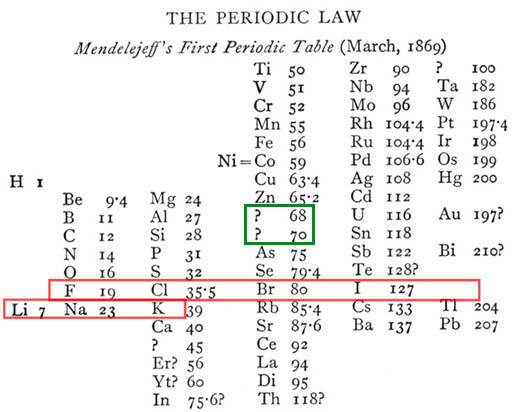# Which atom is it?The electric charge density inside an atomic nucleus of radius $R$ is $\rho (r) = {\rho}_{0} \left[1-{\left(\frac{r}{R}\right)}^{2}\right].$ If ${\rho}_{0} = 5 \times {10}^{25}\text{ C/m}^{3}$ and $R = 3.4 \times {10}^{-15}\text{ m}$, what is the total charge?

Express your answer to the nearest elementary charge (should be an integer).

×